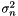#Boost C++ Libraries

...one of the most highly regarded and expertly designed C++ library projects in the world.

This is the documentation for an old version of boost. Click here for the latest Boost documentation.

## Struct template immediate_rolling_variance_impl

boost::accumulators::impl::immediate_rolling_variance_impl — Iterative calculation of the rolling variance.

## Synopsis

```// In header: <boost/accumulators/statistics/rolling_variance.hpp>

template<typename Sample>
struct immediate_rolling_variance_impl {
// types
typedef numeric::functional::fdiv< Sample, std::size_t >::result_type result_type;

// construct/copy/destruct
template<typename Args> immediate_rolling_variance_impl(Args const &);

// public member functions
template<typename Args> void operator()(Args const &);
template<typename Args> result_type result(Args const &) const;

// private member functions
template<typename T>
void prevent_underflow(T &,
typename boost::enable_if< boost::is_arithmetic< T >, T >::type * = 0);
template<typename T>
void prevent_underflow(T &,
typename boost::disable_if< boost::is_arithmetic< T >, T >::type * = 0);
};```

## Description

Iterative calculation of sample varianceis done as follows, see also http://en.wikipedia.org/wiki/Algorithms_for_calculating_variance. For a rolling window of size, for the firstsamples, the variance is computed according to the formula

Equation 1.13.where the sum of squarescan be recursively computed as:

Equation 1.14.and the estimate of the sample mean as:

Equation 1.15.For further samples, when the rolling window is fully filled with data, one has to take into account that the oldest sampleis dropped from the window. The sample variance over the window now becomes:

Equation 1.16.where the sum of squaresnow equals:

Equation 1.17.and the estimated mean is:

Equation 1.18.Note that the sample variance is not defined for.

### `immediate_rolling_variance_impl` public construct/copy/destruct

1. `template<typename Args> immediate_rolling_variance_impl(Args const & args);`

### `immediate_rolling_variance_impl` public member functions

1. `template<typename Args> void operator()(Args const & args);`
2. `template<typename Args> result_type result(Args const & args) const;`

### `immediate_rolling_variance_impl` private member functions

1. ```template<typename T>
void prevent_underflow(T & non_negative_number,
typename boost::enable_if< boost::is_arithmetic< T >, T >::type * = 0);```
2. ```template<typename T>
void prevent_underflow(T & non_arithmetic_quantity,
typename boost::disable_if< boost::is_arithmetic< T >, T >::type * = 0);```
 Copyright © 2005, 2006 Eric Niebler Distributed under the Boost Software License, Version 1.0. (See accompanying file LICENSE_1_0.txt or copy at http://www.boost.org/LICENSE_1_0.txt)